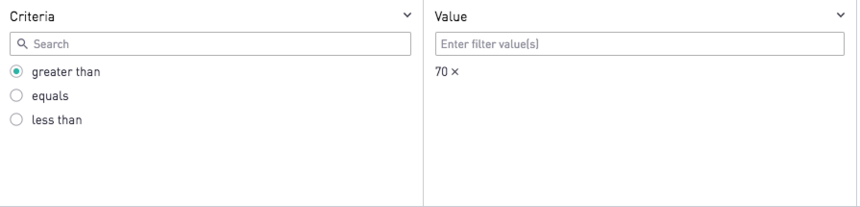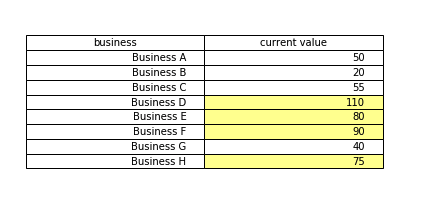Dynamic Conditional Formatting with Periscope Filters and Python

Filters are a useful way to hone in on a specific subset of data, but let's say that you prefer highlighting records that meet the filtering criteria, rather than eliminating others from view entirely. This is definitely possible with Periscope Data's R/Python integration.

First, we need to create a couple filters. Once for the criteria (greater than, less than, equals), and another for the threshold value. Here, my Criteria filter is created from the "Type in names and values" option, and the Value filter is a free input filter ("Allow users to enter filter values")Then, these filter values need to be passed in as columns in the SQL output of our query. For instance, say our SQL query looks like this:

select
,"current value"
from table

Now, we want the query to read:

select
, "current value"
,'[criteria|equals]' as criteria
,[value|0] as val
from table

Notice how here we are using direct replacement filters with default pipe notation. This allows us to handle cases where no filter values are selected.

Now that the filter inputs are passed in via SQL, it's time to use Python to create our table in Python and apply highlights where appropriate:

# SQL output is imported as a pandas dataframe variable called "df"
import matplotlib.pyplot as plt
import pandas as pd
from pandas.tools.plotting import table

#Source 1: https://stackoverflow.com/questions/35634238/how-to-save-a-pandas-dataframe-table-as-a-png
#Source 2: https://stackoverflow.com/questions/46663911/how-to-assign-specific-colors-to-specific-cells-in-a-matplotlib-table

df2=df[df.columns[:2]]
ax = plt.subplot(111, frame_on=False)
ax.xaxis.set_visible(False)
ax.yaxis.set_visible(False)

the_table=table(ax, df2, rowLabels=['']*df2.shape, bbox=[0,0.25,1,0.5])
ax.axis('tight')

if df["criteria"]=='equals':
for i in range(df2.shape):
if df2["current value"][i]==df["val"][i]:
the_table._cells[(i+1, 1)].set_facecolor("#ffff99")
elif df["criteria"]=='greater than':
for i in range(df2.shape):
if df2["current value"][i]>df["val"][i]:
the_table._cells[(i+1, 1)].set_facecolor("#ffff99")
else:
for i in range(df2.shape):
if df2["current value"][i]<df["val"][i]:
the_table._cells[(i+1, 1)].set_facecolor("#ffff99")

# Use Periscope to visualize a dataframe or an image by passing data to periscope.output()
periscope.output(plt)

For the below image, my criteria is "greater than" and my value is 70